# Conduction: One Dimensional (Part - 4) Chemical Engineering Notes | EduRev

## Chemical Engineering : Conduction: One Dimensional (Part - 4) Chemical Engineering Notes | EduRev

The document Conduction: One Dimensional (Part - 4) Chemical Engineering Notes | EduRev is a part of the Chemical Engineering Course Heat Transfer for Engg..
All you need of Chemical Engineering at this link: Chemical Engineering

Lecture 6 - Conduction: One Dimensional, Heat Transfer

2.3 Steady-state heat conduction through a variable area
It was observed in the previous discussion that for the given plane wall the area for heat transfer was constant along the heat flow direction. The plane solid wall was one of the geometries but if we take some other geometry (tapered plane, cylindrical body, spherical body etc.) in which the area changes in the direction of heat flow. Now we will consider geometrical configuration which will be mathematically simple and also of great engineering importance like hollow cylinder and hollow sphere. In these cases the heat transfer area varies in the radial direction of heat conduction. We will take up both the cases one by one in the following sections.

2.3.1 Cylinder
Consider a hollow cylinder as shown in the fig.2.9a. The inner and outer radius is represented by rand r, whereas Ti and To (Ti > T) represent the uniform temperature of the inner and outer wall, respectively.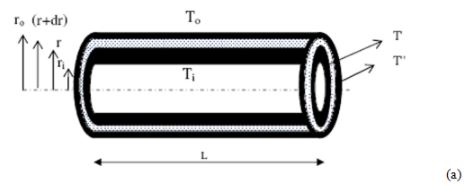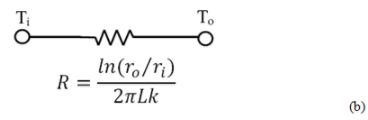Fig. 2.9. (a) Hollow cylinder, (b) equivalent electrical circuit

Consider a very thin hollow cylinder of thickness dr in the main geometry (fig.2.9a) at a radial distance r. If dr is small enough with respect to r, then the area of the inner and outer surface of the thin cylinder may be considered to be of same area. In other words, for very small dr with respect to r, the lines of heat flow may be considered parallel through the differential element in radial outward direction.

We may ignore the heat flow through the ends if the cylinder is sufficiently large. We may thus eliminate any dependence of the temperature on the axial coordinate and for one dimensional steady state heat conduction, the rate of heat transfer for the thin cylinder,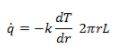Where dT is the temperature difference between the inner and outer surface of the thin cylinder considered above and k is the thermal conductivity of the cylinder.
On rearranging,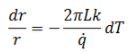To get the heat flow through the thick wall cylinder, the above equation can be integrated between the limits,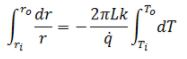On solving,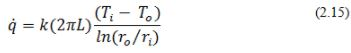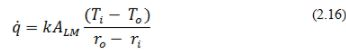Where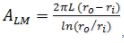, and the careful analysis of the above equation shows that the expression is same as for heat flow through the plane wall of thickness (ro–ri ) except the expression for the area. The ALM is known as log mean area of the cylinder, whose length is L and radius is rLM (=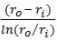). The fig.2.9b shows the equivalent electrical circuit of the fig.2.9b.

Now we have learnt that how to represent the analogous electrical circuit for the cylindrical case. It will provide the building block for the composite cylinders similar to the plane composite we have learnt earlier. The following fig.2.10a shows a composite cylinder with 4-layers of solid material of different inner and outer diameter as well as thermal conductivity. The equivalent electrical circuit is shown below in fig.2.10b.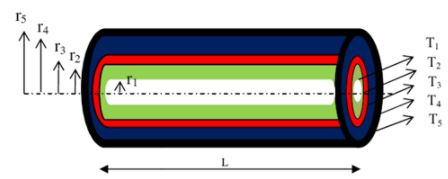(a)

###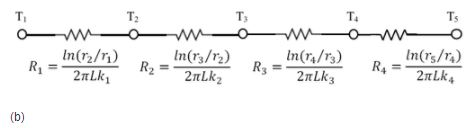Fig.2.10.(a) Four layer composite hollow cylinder, (a) equivalent electrical circuit

The total heat transfer at steady-state will be,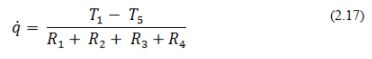where R1 , R, R3 , and R4 are represented in the fig.2.10b.

2.3.2 Sphere
The rate of heat transfer through a hollow sphere can be determined in a similar manner as for cylinder. The students are advised to derive the following expression shown below.

The final expression for the rate of heat flow is,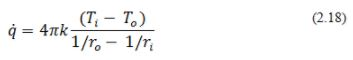Offer running on EduRev: Apply code STAYHOME200 to get INR 200 off on our premium plan EduRev Infinity!

## Heat Transfer for Engg.

46 videos|60 docs|59 tests

,

,

,

,

,

,

,

,

,

,

,

,

,

,

,

,

,

,

,

,

,

;